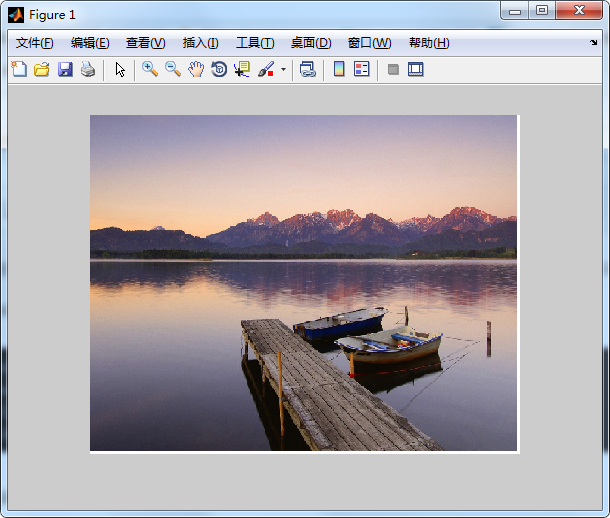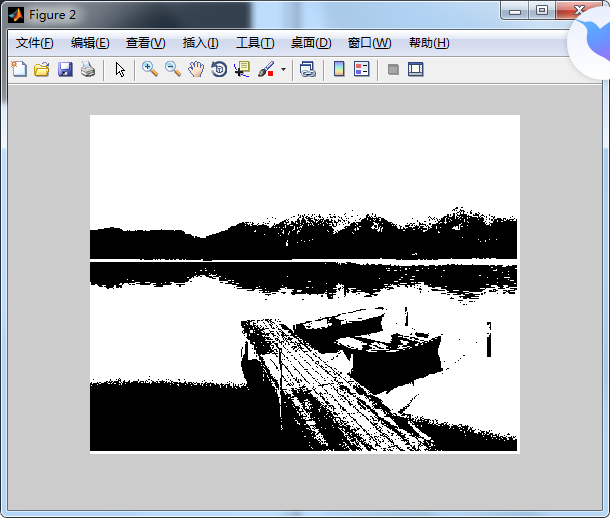# 图像处理阈值变换

## [Python图像处理] 七.图像阈值化处理及算法对比

2018-10-30 22:19:36 Eastmount 阅读数 8399
• ###### matlab 基于阈值分割的区域生长的医学图像分割3讲

matlab 基于阈值分割的区域生长的医学图像分割3讲 </p>

3课时 23分钟 614人学习 刘昱显
免费试看

PS：请求帮忙点个Star，哈哈，第一次使用Github，以后会分享更多代码，一起加油。

1.阈值化
2.二进制阈值化
3.反二进制阈值化
4.截断阈值化
5.反阈值化为0
6.阈值化为0

PS：文章参考自己以前系列图像处理文章及OpenCV库函数，同时部分参考网易云视频，推荐大家去学习。同时，本篇文章涉及到《计算机图形学》基础知识，请大家下来补充。

PSS：2019年1~2月作者参加了CSDN2018年博客评选，希望您能投出宝贵的一票。我是59号，Eastmount，杨秀璋。投票地址：https://bss.csdn.net/m/topic/blog_star2018/index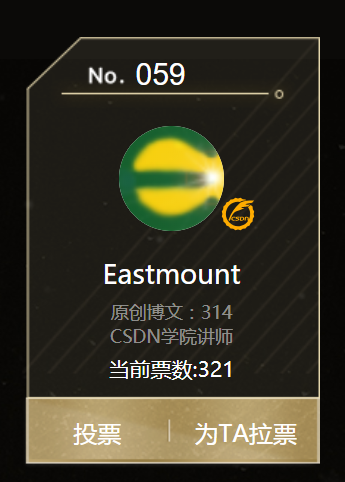# 一. 阈值化

（注：该部分参考作者的论文《基于苗族服饰的图像锐化和边缘提取技术研究》）

$\begin{cases} Y=0，gray=T\\ \end{cases}$

Python OpenCV中提供了阈值函数threshold()实现二值化处理，其公式及参数如下图所示：
retval, dst = cv2.threshold(src, thresh, maxval, type)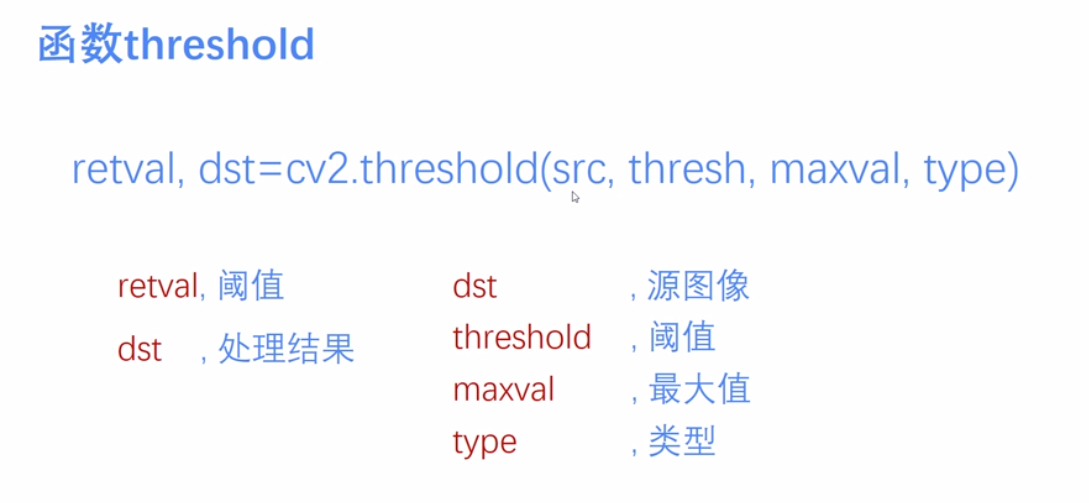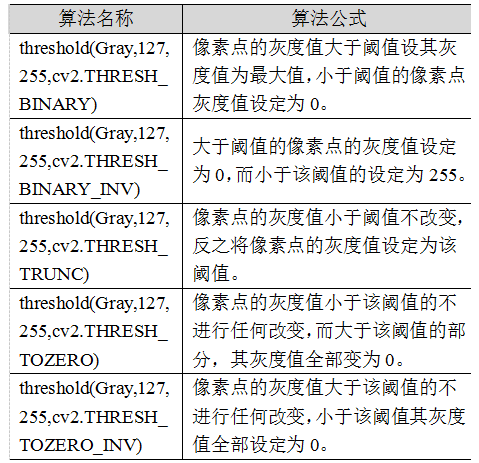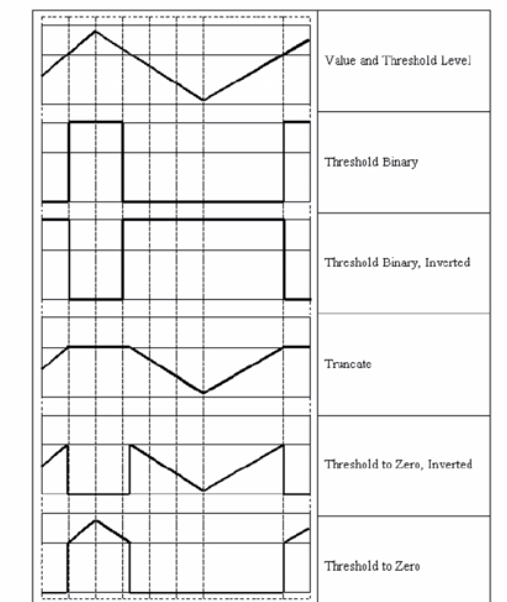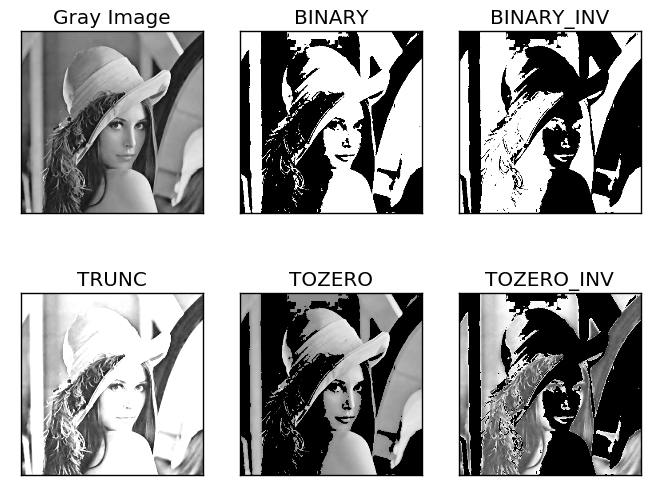# 二. 二进制阈值化

$dst(x,y) = \begin{cases} maxVal， if src(x,y)>thresh\\ 0，otherwise\\ \end{cases}$
(1) 大于等于127的像素点的灰度值设定为最大值（如8位灰度值最大为255）
(2) 灰度值小于127的像素点的灰度值设定为0

#encoding:utf-8
import cv2
import numpy as np

#读取图片

#灰度图像处理
GrayImage = cv2.cvtColor(src,cv2.COLOR_BGR2GRAY)

#二进制阈值化处理
r, b = cv2.threshold(GrayImage, 127, 255, cv2.THRESH_BINARY)
print r

#显示图像
cv2.imshow("src", src)
cv2.imshow("result", b)

#等待显示
cv2.waitKey(0)
cv2.destroyAllWindows()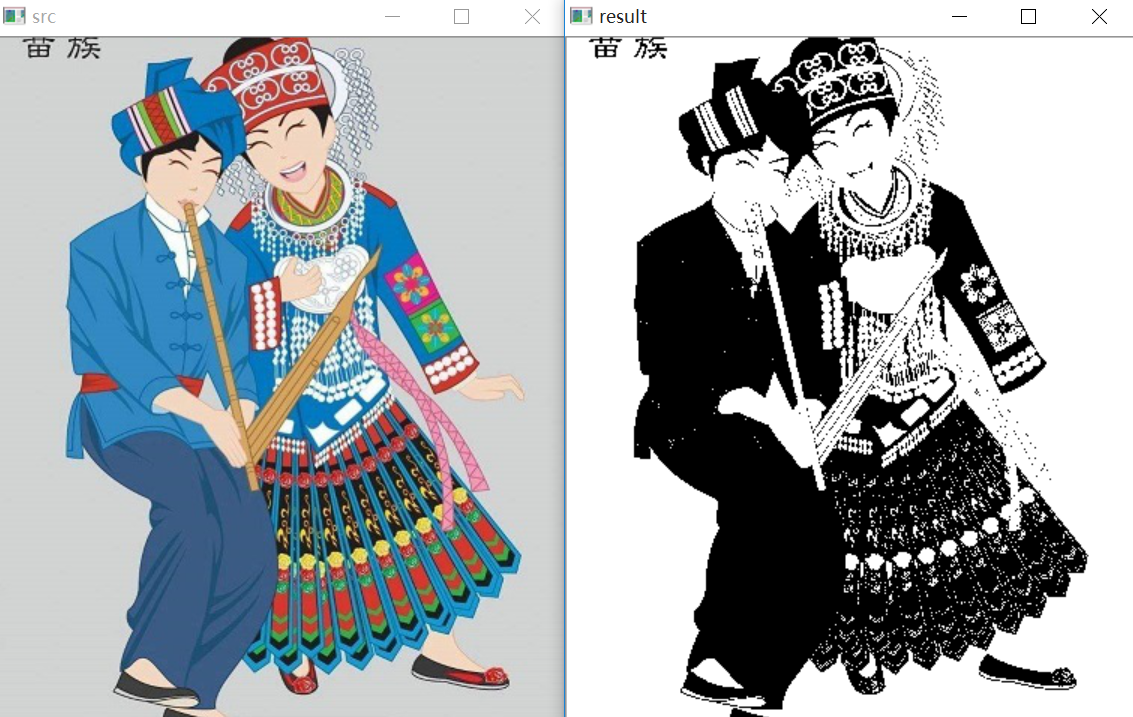# 三. 反二进制阈值化

$dst(x,y) = \begin{cases} 0， if src(x,y)>thresh\\ maxVal，otherwise\\ \end{cases}$
(1) 大于127的像素点的灰度值设定为0（以8位灰度图为例）
(2) 小于该阈值的灰度值设定为255

#encoding:utf-8
import cv2
import numpy as np

#读取图片

#灰度图像处理
GrayImage = cv2.cvtColor(src,cv2.COLOR_BGR2GRAY)

#反二进制阈值化处理
r, b = cv2.threshold(GrayImage, 127, 255, cv2.THRESH_BINARY_INV)
print r

#显示图像
cv2.imshow("src", src)
cv2.imshow("result", b)

#等待显示
cv2.waitKey(0)
cv2.destroyAllWindows()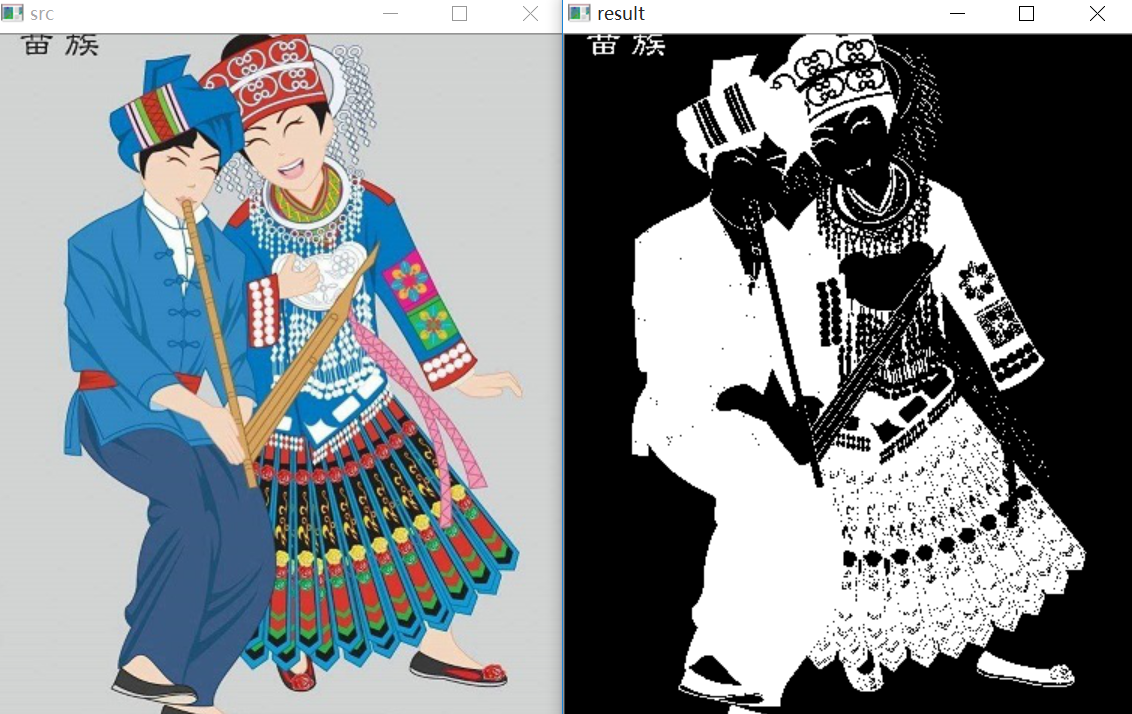# 四. 截断阈值化

$dst(x,y) = \begin{cases} threshold， if src(x,y)>thresh\\ src(x,y)，otherwise\\ \end{cases}$
(1) 大于等于127的像素点的灰度值设定为该阈值127
(2) 小于该阈值的灰度值不改变

#encoding:utf-8
import cv2
import numpy as np

#读取图片

#灰度图像处理
GrayImage = cv2.cvtColor(src,cv2.COLOR_BGR2GRAY)

#截断阈值化处理
r, b = cv2.threshold(GrayImage, 127, 255, cv2.THRESH_TRUNC)
print r

#显示图像
cv2.imshow("src", src)
cv2.imshow("result", b)

#等待显示
cv2.waitKey(0)
cv2.destroyAllWindows()# 五. 反阈值化为0

$dst(x,y) = \begin{cases} 0， if src(x,y)>thresh\\ src(x,y)，otherwise\\ \end{cases}$
(1) 大于等于阈值127的像素点变为0
(2) 小于该阈值的像素点值保持不变

#encoding:utf-8
import cv2
import numpy as np

#读取图片

#灰度图像处理
GrayImage = cv2.cvtColor(src,cv2.COLOR_BGR2GRAY)

#反阈值化为0处理
r, b = cv2.threshold(GrayImage, 127, 255, cv2.THRESH_TOZERO_INV)
print r

#显示图像
cv2.imshow("src", src)
cv2.imshow("result", b)

#等待显示
cv2.waitKey(0)
cv2.destroyAllWindows()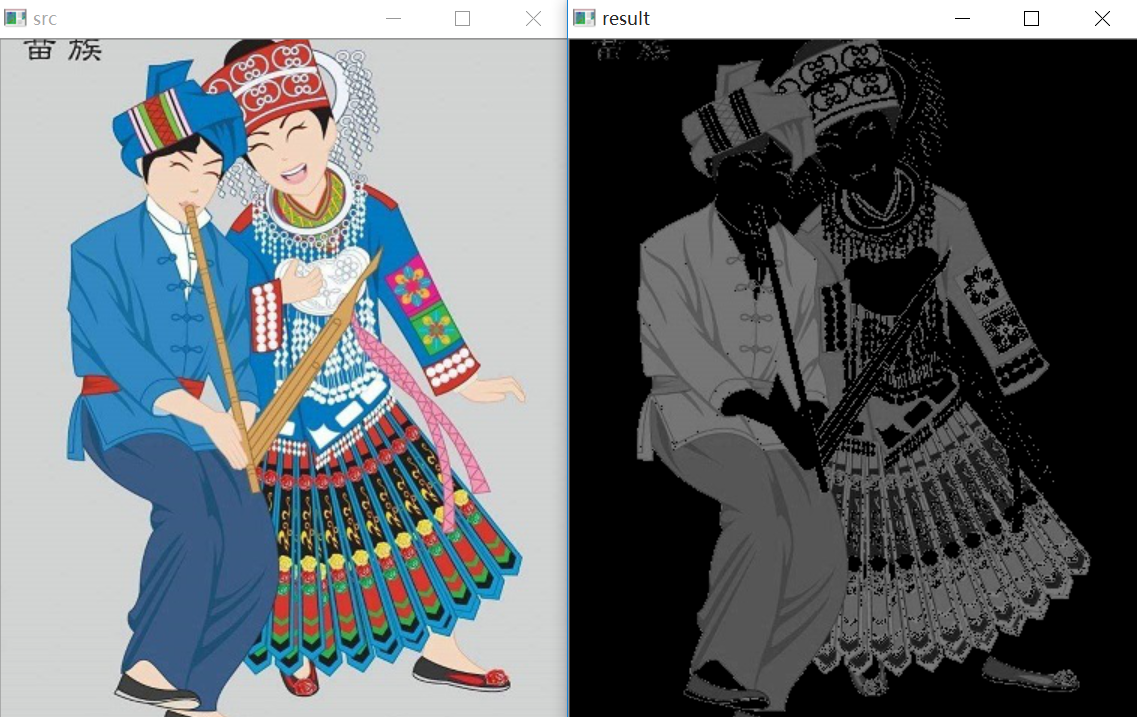# 六. 阈值化为0

$dst(x,y) = \begin{cases} src(x,y)， if src(x,y)>thresh\\ 0，otherwise\\ \end{cases}$
(1) 大于等于阈值127的像素点，值保持不变
(2) 小于该阈值的像素点值设置为0

#encoding:utf-8
import cv2
import numpy as np

#读取图片

#灰度图像处理
GrayImage = cv2.cvtColor(src,cv2.COLOR_BGR2GRAY)

#阈值化为0处理
r, b = cv2.threshold(GrayImage, 127, 255, cv2.THRESH_TOZERO)
print r

#显示图像
cv2.imshow("src", src)
cv2.imshow("result", b)

#等待显示
cv2.waitKey(0)
cv2.destroyAllWindows()#encoding:utf-8
import cv2
import numpy as np
import matplotlib.pyplot as plt

#读取图像
lenna_img = cv2.cvtColor(img,cv2.COLOR_BGR2RGB)
GrayImage=cv2.cvtColor(img,cv2.COLOR_BGR2GRAY)

#阈值化处理
ret,thresh1=cv2.threshold(GrayImage,127,255,cv2.THRESH_BINARY)
ret,thresh2=cv2.threshold(GrayImage,127,255,cv2.THRESH_BINARY_INV)
ret,thresh3=cv2.threshold(GrayImage,127,255,cv2.THRESH_TRUNC)
ret,thresh4=cv2.threshold(GrayImage,127,255,cv2.THRESH_TOZERO)
ret,thresh5=cv2.threshold(GrayImage,127,255,cv2.THRESH_TOZERO_INV)

#显示结果
titles = ['Gray Image','BINARY','BINARY_INV','TRUNC','TOZERO','TOZERO_INV']
images = [GrayImage, thresh1, thresh2, thresh3, thresh4, thresh5]
for i in xrange(6):
plt.subplot(2,3,i+1),plt.imshow(images[i],'gray')
plt.title(titles[i])
plt.xticks([]),plt.yticks([])
plt.show()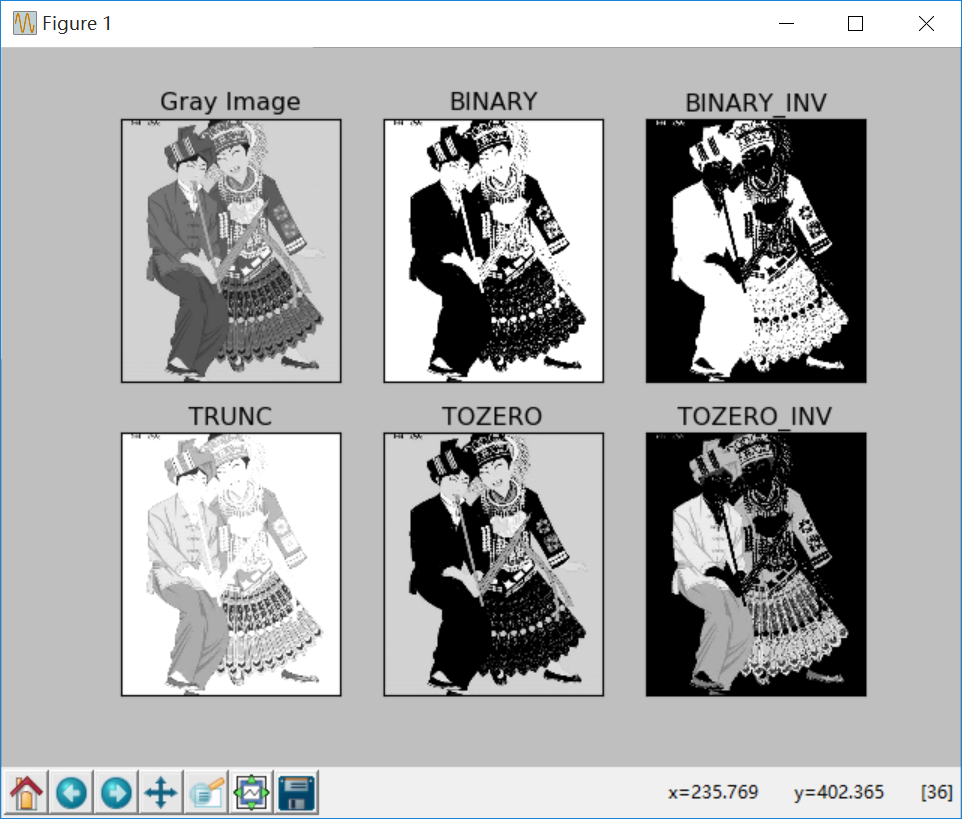（By：Eastmount 2018-10-30 晚上10点 https://blog.csdn.net/Eastmount/）

## 数字图像处理（三）灰度线性变化、非线性变化、阈值化、均衡化

2018-05-22 15:53:12 hjxu2016 阅读数 8671
• ###### matlab 基于阈值分割的区域生长的医学图像分割3讲

matlab 基于阈值分割的区域生长的医学图像分割3讲 </p>

3课时 23分钟 614人学习 刘昱显
免费试看

## 一. 点运算与初始操作

图像的点运算是图像处理中非常基础的技术，它主要用于改变一篇图像的灰度分布范围，通过一定的变换函数将图像的像素进行转换，最终生成一幅新的图像。点运算的最大特点就是输出像素值只与当前输入像素值相关。定义如下。
点运算(Point Operation)指对于一幅输入图像，将产生一幅输出图像，输出图像的每个像素点的灰度值由输入像素点决定。
点运算由灰度变换函数(Grap Scale Transformation，GST)确定：B(x,y)=F[A(x,y)]

需要注意一下几点：
(1).与局部或邻域运算的差别，输入像素和输出像素是一一对应的；(2).与几何运算的差别，不改变图像的空间关系；(3).又称为对比增强，对比拉伸或灰度变换。

在前面第四篇博客的基础上增加点运算处理。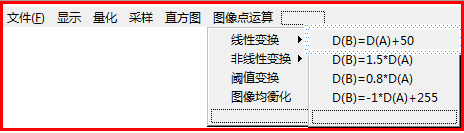对应的ID值为：
线性变换 ID_DYS_XXYD(点运算 线性移动) ID_DYS_XXZQ( 点运算 线性增强)
ID_DYS_XXJX(点运算 线性减小)  ID_DYS_XXQB(点运算 线性求补)
非线性变换 ID_DYS_FXXPF(点运算 非线性平方) ID_DYS_FXXHS(非线性函数)
阈值变换 ID_DYS_YZBH(点运算 阈值变换) 图像均衡化 ID_DYS_JHH

第二步：打开类向导(Ctrl+W)，为点运算每个ID菜单添加相应的功能处理函数，如下图所示：选择类CImageProcessingView，在选择IDs为ID_DYS_...(点运算)添加函数OnDysXxqb()线性求补。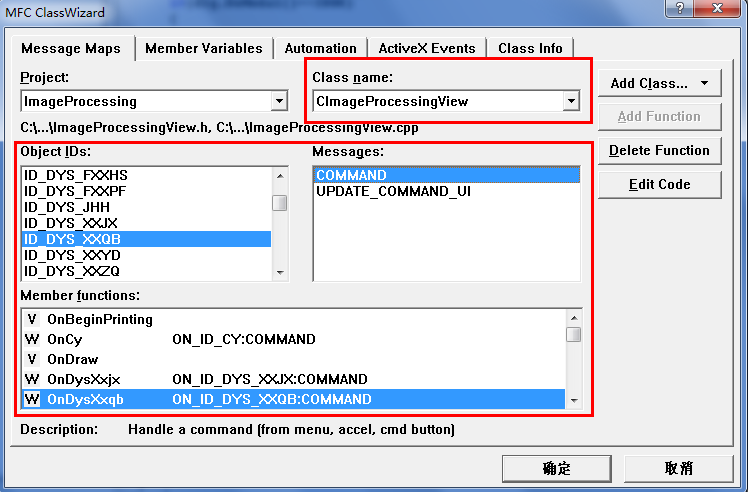## 二. 线性变换

图像线性变换是通过建立灰度映射来调整资源图像的灰度，从而达到图像增强的目的。其中GST函数f(D)为线性的，即：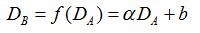若a=1,b=0图像像素不发生变化
若a=1,b!=0图像所有灰度值上移或下移
若a>1输出图像对比度增强
若0<a<1输出图像对比度减小
若a<0暗区域变亮，亮区域变暗，图像求补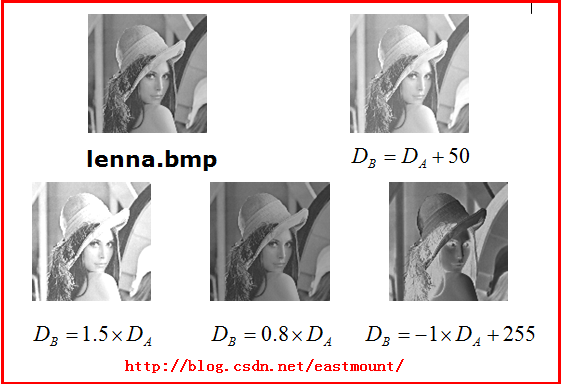1.D(B)=D(A)+50
首先是图像移动，代码如下：
[cpp] view plain
1. /**********************************************************************/
2. /* 图像点运算 4种线性变化直方图：
3. /* ID_DYS_XXYD:表示线性灰度变化移动 D(B)=D(A)+50  灰度值上移下移
4. /* ID_DYS_XXZQ:表示线性灰度变化增强 D(B)=1.5*D(A) 图像对比度增强
5. /* ID_DYS_XXJX:表示线性灰度变化减小 D(B)=0.8*D(A) 图像对比度减小
6. /* ID_DYS_XXQB:表示线性灰度求补 D(B)=-1*D(A)+255  图像暗区变亮,亮区变暗
7. /**********************************************************************/
8.
9. // 1.点运算 线性灰度变化移动 D(B)=D(A)+50
10. void CImageProcessingView::OnDysXxyd()
11. {
12.     // TODO: Add your command handler code here
13.     if(numPicture==0) {
14.         AfxMessageBox("载入图片后才能线性灰度运算!",MB_OK,0);
15.         return;
16.     }
17.     AfxMessageBox("线性灰度直方图-灰度变化移动 D(B)=D(A)+50!",MB_OK,0);
18.     int i;
19.     //打开临时的图片
20.     FILE *fpo = fopen(BmpName,"rb");
21.     FILE *fpw = fopen(BmpNameLin,"wb+");
22.     //读取文件
27.     //灰度图像
28.     unsigned char color;
29.     unsigned char red,green,blue;
30.     for( i=0; i<m_nImage/3; i++ )
31.     {
35.
36.         if( (int)red+50 >255 )
37.             red=255;
38.         else
39.             red=(int)red+50;
40.
41.         if( (int)green+50>255 )
42.             green=255;
43.         else
44.             green=(int)green+50;
45.
46.         if( (int)blue+50>255 )
47.             blue=255;
48.         else
49.             blue=(int)blue+50;
50.
51.         fwrite(&red,sizeof(char),1,fpw);
52.         fwrite(&green,sizeof(char),1,fpw);
53.         fwrite(&blue,sizeof(char),1,fpw);
54.     }
55.     fclose(fpo);
56.     fclose(fpw);
57.     numPicture = 2;
58.     level=101;       //赋值101在ShowBitmap中调用显示处理后的图片
59.     Invalidate();
60. }

同时修改void CImageProcessingView::ShowBitmap(CDC *pDC,
CString BmpName)函数中的代码：

[cpp] view plain
1. else        //图像点运算 线性变化
2. if(level=101)
3. {
6. }
运行效果如下图所示，同时我截取了直方图(RGB相同只显示一种)。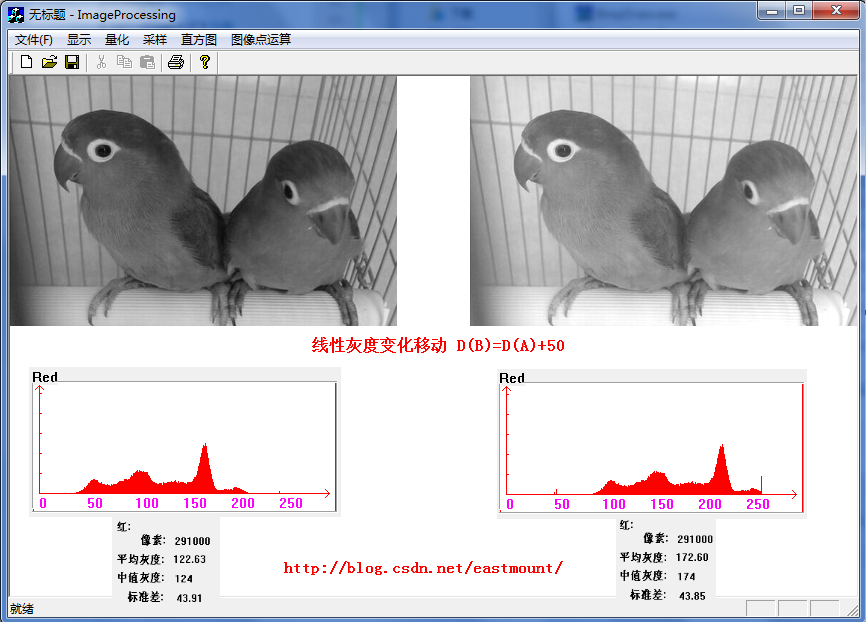可以发现图像的灰度上移了50，图像更白了（黑0-255白）。

2.D(B)=1.5*D(A)
[cpp] view plain
1. // 2.点运算 线性灰度变化增强 D(B)=1.5*D(A)
2. void CImageProcessingView::OnDysXxzq()
3. {
4.     if(numPicture==0) {
5.         AfxMessageBox("载入图片后才能线性灰度运算!",MB_OK,0);
6.         return;
7.     }
8.     AfxMessageBox("线性灰度直方图-灰度变化增强 D(B)=1.5*D(A)!",MB_OK,0);
9.     int i;
10.     //打开临时的图片
11.     FILE *fpo = fopen(BmpName,"rb");
12.     FILE *fpw = fopen(BmpNameLin,"wb+");
17.     //灰度图像
18.     unsigned char color;
19.     unsigned char red,green,blue;
20.     for( i=0; i<m_nImage/3; i++ )
21.     {
25.
26.         if( (int)red*1.5 >255 )
27.             red=255;
28.         else
29.             red=(int)red*1.5;
30.
31.         if( (int)green*1.5>255 )
32.             green=255;
33.         else
34.             green=(int)green*1.5;
35.
36.         if( (int)blue*1.5>255 )
37.             blue=255;
38.         else
39.             blue=(int)blue*1.5;
40.
41.         fwrite(&red,sizeof(char),1,fpw);
42.         fwrite(&green,sizeof(char),1,fpw);
43.         fwrite(&blue,sizeof(char),1,fpw);
44.     }
45.     fclose(fpo);
46.     fclose(fpw);
47.     numPicture = 2;
48.     level=101;      //线性变化 ShowBitmap中调用
49.     Invalidate();
50. }
运行效果如下图所示，图像对比度增强，平均灰度122*1.5=181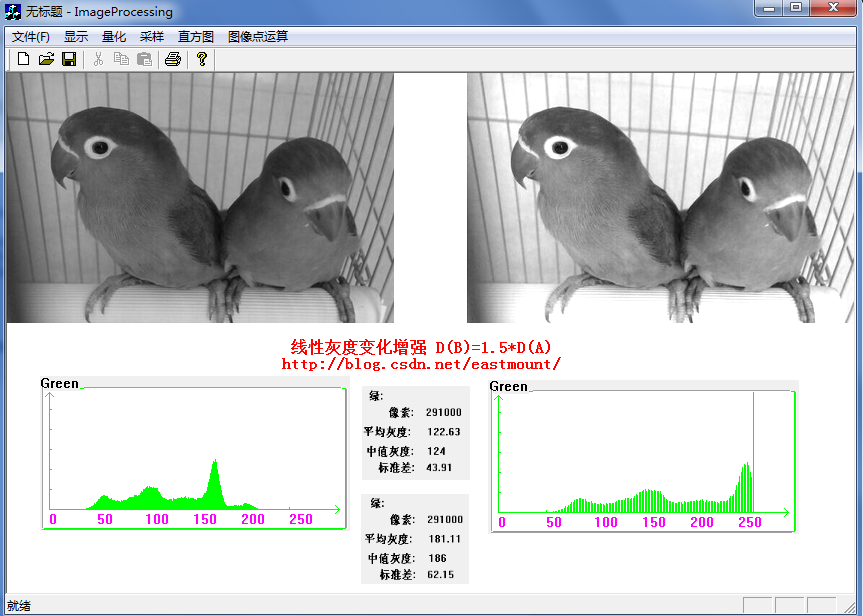3.D(B)=0.8*D(A)
[cpp] view plain
1. // 3.点运算 线性灰度变化减小D(B)=0.8*D(A)
2. void CImageProcessingView::OnDysXxjx()
3. {
4.     if(numPicture==0) {
5.         AfxMessageBox("载入图片后才能线性灰度处理!",MB_OK,0);
6.         return;
7.     }
8.     AfxMessageBox("线性灰度直方图-灰度减小 D(B)=0.8*D(A)!",MB_OK,0);
9.     int i;
10.     //打开临时的图片
11.     FILE *fpo = fopen(BmpName,"rb");
12.     FILE *fpw = fopen(BmpNameLin,"wb+");
17.     //灰度图像
18.     unsigned char color;
19.     unsigned char red,green,blue;
20.     for( i=0; i<m_nImage/3; i++ )
21.     {
25.
26.         red=(int)red*0.8;
27.         green=(int)green*0.8;
28.         blue=(int)blue*0.8;
29.
30.         fwrite(&red,sizeof(char),1,fpw);
31.         fwrite(&green,sizeof(char),1,fpw);
32.         fwrite(&blue,sizeof(char),1,fpw);
33.     }
34.     fclose(fpo);
35.     fclose(fpw);
36.     numPicture = 2;
37.     level=101;
38.     Invalidate();
39. }
运行如下图所示，图像减弱。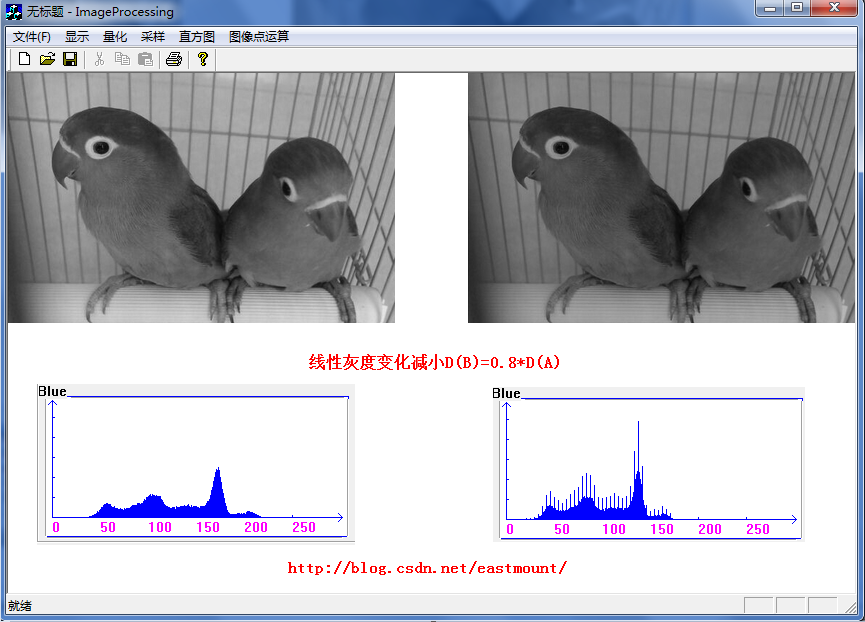4.D(B)=-1*D(A)+255
[cpp] view plain
1. // 4.点运算 线性灰度求补 D(B)=-1*D(A)+255
2. void CImageProcessingView::OnDysXxqb()
3. {
4.     if(numPicture==0) {
5.         AfxMessageBox("载入图片后才能线性灰度处理!",MB_OK,0);
6.         return;
7.     }
8.     AfxMessageBox("线性灰度直方图-灰度求补 D(B)=-1*D(A)+255!",MB_OK,0);
9.     int i;
10.     //打开临时的图片
11.     FILE *fpo = fopen(BmpName,"rb");
12.     FILE *fpw = fopen(BmpNameLin,"wb+");
17.     //灰度图像
18.     unsigned char color;
19.     unsigned char red,green,blue;
20.     for( i=0; i<m_nImage/3; i++ )
21.     {
25.
26.         red=(int)red*(-1)+255;
27.         green=(int)green*(-1)+255;
28.         blue=(int)blue*(-1)+255;
29.
30.         fwrite(&red,sizeof(char),1,fpw);
31.         fwrite(&green,sizeof(char),1,fpw);
32.         fwrite(&blue,sizeof(char),1,fpw);
33.     }
34.     fclose(fpo);
35.     fclose(fpw);
36.     numPicture = 2;
37.     level=101;
38.     Invalidate();
39. }
运行效果如下图所示，它是图像的求补，发现直方图是互补的。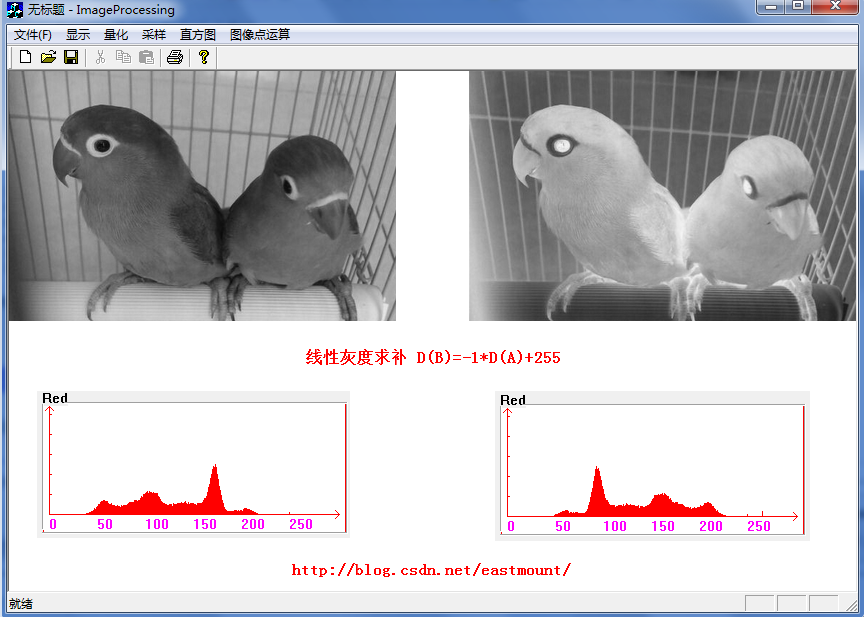PS:注意图片下面的直方图应该还有一个处理后的直方图，但原理都一样，我不想重复工作，你自己可以去简单实现下，参考第四篇文章。同时这些图片制作还挺麻烦的，只是为了给你更好的呈现它们的变化，希望对你有用和尊重作者，不喜勿喷~

## 三. 非线性变换

灰度非线性变换主要包括对数变换、幂次变换、指数变换、分段函数变换，通过非线性关系对图像进行灰度处理，下面主要讲解课件中的两个函数对其进行处理。其中对数变换实现了扩展低灰度值而压缩高灰度值的效果，图像灰度分布更符合而你的视觉特征。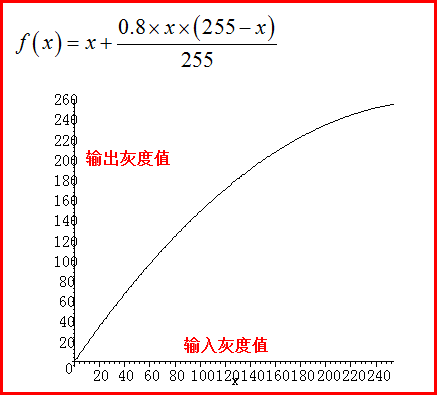1.D(B)=D(A)*D(A)/252
[cpp] view plain
1. /************************************************************************/
2. /* 2种非线性变化直方图：
3. /* ID_DYS_FXXPF:表示非线性平方灰度变化,D(B)=D(A)*D(A)/255
4. /* ID_DYS_FXXHS:表示非线性函数灰度变化,D(B)=D(A)+0.8*D(A)*(255-D(A))/255
5. /************************************************************************/
6.
7. // 非线性平方灰度变化 D(B)=D(A)*D(A)/252
8. void CImageProcessingView::OnDysFxxpf()
9. {
10.     if(numPicture==0)
11.     {
12.         AfxMessageBox("载入图片后才能非线性灰度处理!",MB_OK,0);
13.         return;
14.     }
15.     AfxMessageBox("非线性灰度变化 D(B)=D(A)*D(A)/255!",MB_OK,0);
16.     int i;
17.     //打开临时的图片
18.     FILE *fpo = fopen(BmpName,"rb");
19.     FILE *fpw = fopen(BmpNameLin,"wb+");
20.     //读取文件
25.     //灰度图像
26.     unsigned char color;
27.     unsigned char red,green,blue;
28.     for( i=0; i<m_nImage/3; i++ )
29.     {
33.
34.         red=(int)red*(int)red/255;
35.         green=(int)green*(int)green/255;
36.         blue=(int)blue*(int)blue/255;
37.
38.         fwrite(&red,sizeof(char),1,fpw);
39.         fwrite(&green,sizeof(char),1,fpw);
40.         fwrite(&blue,sizeof(char),1,fpw);
41.     }
42.     fclose(fpo);
43.     fclose(fpw);
44.     numPicture = 2;
45.     level=101;
46.     Invalidate();
47. }
运行效果如下图所示：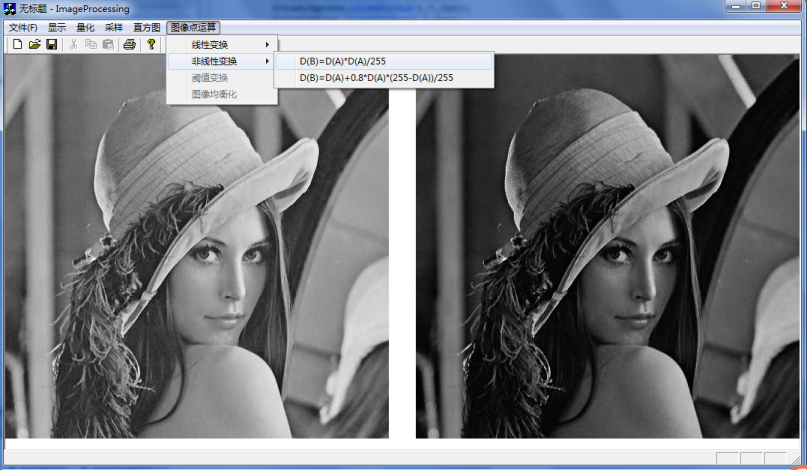2.D(B)=D(A)+0.8*D(A)*(255-D(A))/255
[cpp] view plain
1. // 非线性函数灰度变化 D(B)=D(A)+0.8*D(A)*(255-D(A))/255
2. void CImageProcessingView::OnDysFxxhs()
3. {
4.     if(numPicture==0)
5.     {
6.         AfxMessageBox("载入图片后才能非线性灰度处理!",MB_OK,0);
7.         return;
8.     }
9.     AfxMessageBox("线性灰度直方图-灰度变化增强 D(B)=D(A)+0.8*D(A)*(255-D(A))/255!",MB_OK,0);
10.     int i;
11.
12.     FILE *fpo = fopen(BmpName,"rb");
13.     FILE *fpw = fopen(BmpNameLin,"wb+");
18.
19.     unsigned char color;
20.     unsigned char red,green,blue;
21.     for( i=0; i<m_nImage/3; i++ )
22.     {
26.
27.         if( ((int)red+0.8*(int)red*(255-(int)red)/255) > 255 )
28.             red=255;
29.         else
30.             red=(int)red+0.8*(int)red*(255-(int)red)/255;
31.
32.         if( ((int)green+0.8*(int)green*(255-(int)green)/255) > 255 )
33.             green=255;
34.         else
35.             green=(int)green+0.8*(int)green*(255-(int)green)/255;
36.
37.         if( ((int)blue+0.8*(int)blue*(255-(int)blue)/255) > 255 )
38.             blue=255;
39.         else
40.             blue=(int)blue+0.8*(int)blue*(255-(int)blue)/255;
41.
42.         fwrite(&red,sizeof(char),1,fpw);
43.         fwrite(&green,sizeof(char),1,fpw);
44.         fwrite(&blue,sizeof(char),1,fpw);
45.     }
46.     fclose(fpo);
47.     fclose(fpw);
48.     numPicture = 2;
49.     level=101;
50.     Invalidate();
51. }
运行效果如下图所示：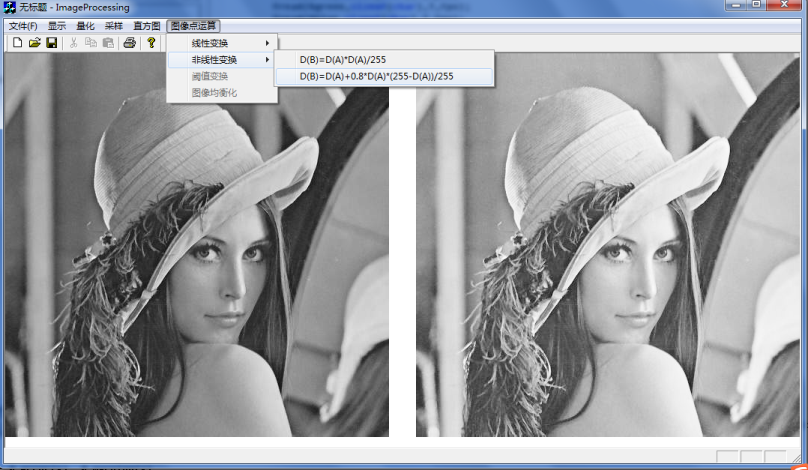写到此处你会发现图像灰度的线性变换和非线性变换是非常简单的，主要是通过以下步骤完成：
第一步：赋值处理后图像的BMP头信息
FILE *fpo = fopen(BmpName,"rb");
FILE *fpw = fopen(BmpNameLin,"wb+");

第二步：通过循环和线性变换或非线性便函函数处理每一个像素
for( i=0; i<m_nImage/3; i++ )
{
处理像素RBG 如:red=(int)red*(int)red/255;
fwrite(&red,sizeof(char),1,fpw);
}

第三步：调用ShowBitmap自定义函数并重绘图像
numPicture = 2;
level=101;
Invalidate();

## 四. 灰度阈值化

阈值又称为临界值，它的目的是确定出一个范围，然后这个范围内的部分使用同一种方法处理，而阈值之外的部分则使用另一种处理方法或保持原样。常用的包括产生二值图：当x<T时y=0，当x>=T时y=255（其中T是阈值）。阈值变换在生物学上的应用比较广泛，常用语细胞图像分割等。
打开类向导(Ctrl+W)生成选择ImageProcessingView类，IDs选择ID_DYS_YZBH后添加相应的函数。代码如下：

[cpp] view plain
1. /**************************************************************/
2. /* ID_DYS_YZBH:表示点运算阈值变换 也看做灰度拉伸
3. /* 此处的拉伸是：阈值化(thresholding)可以看作是削波的一个特例
4. /* 只要令削波中的g1old=g2old就实现了阈值化。
5. /* 阈值就象个门槛，比它大就是白，比它小就是黑,二值
6. /**************************************************************/
7.
8. void CImageProcessingView::OnDysYzbh()
9. {
10.     if(numPicture==0)
11.     {
12.         AfxMessageBox("载入图片后才能点运算阈值化处理!",MB_OK,0);
13.         return;
14.     }
15.     AfxMessageBox("图像点运算阈值化处理!",MB_OK,0);
16.     //读写文件
17.     FILE *fpo = fopen(BmpName,"rb");
18.     FILE *fpw = fopen(BmpNameLin,"wb+");
23.     //处理
24.     unsigned char color;
25.     unsigned char red,green,blue;
26.     for(int i=0; i<m_nImage/3; i++ )
27.     {
31.
32.         if( (int)red > 128 )
33.             red=255;
34.         else
35.             red=0;
36.
37.         if( (int)green > 128 )
38.             green=255;
39.         else
40.             green=0;
41.
42.         if( (int)blue > 128 )
43.             blue=255;
44.         else
45.             blue=0;
46.
47.         fwrite(&red,sizeof(char),1,fpw);
48.         fwrite(&green,sizeof(char),1,fpw);
49.         fwrite(&blue,sizeof(char),1,fpw);
50.     }
51.     fclose(fpo);
52.     fclose(fpw);
53.     numPicture = 2;
54.     level=101;
55.     Invalidate();
56. }
运行效果如下图所示，感觉还挺好看的，显然此时的直方图就是0和255两条直线。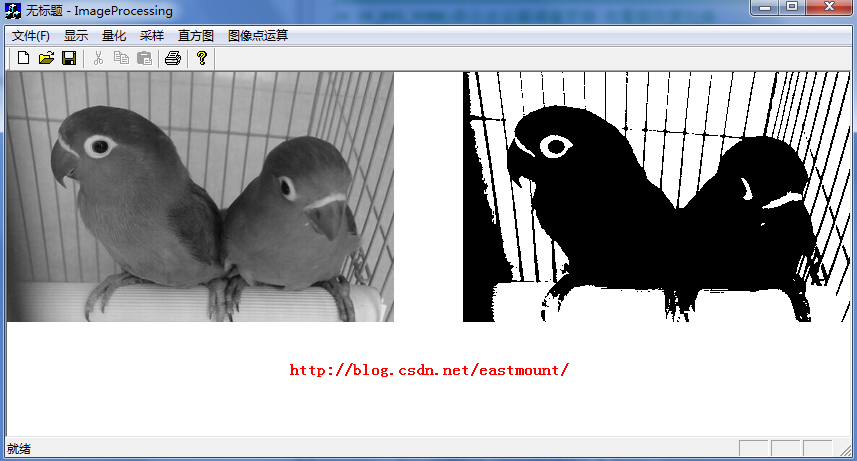## 五. 灰度均衡化

灰度均衡化的目的是使一输入图像转换为在每一灰度级上都有相同的像素点（即输出的直方图是平的），它可以产生一幅灰度级分布概率均衡的图像。
换句话说，经过均衡化后的图像在每一级灰度上像素点的数量相差不大，对应的灰度直方图的每一级高度也相差不大。它是增强图像的有效手段之一。
研究思路是通过直方图变换公式实现：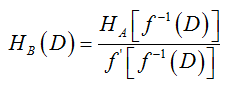它的步骤如下图所示：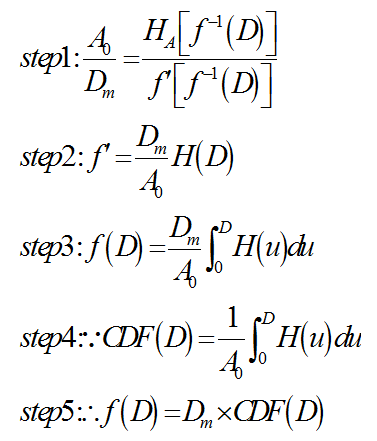例：有一幅图象，共有16级灰度，其直方图分布为Pi, i=0,1,…,15，求经直方图均衡化后，量化级别为10级的灰度图象的直方图分布Qi，其中Pi和Qi为分布的概率，即灰度i出现的次数与总的点数之比。
Pi：0.03, 0, 0.06, 0.10, 0.20, 0.11, 0, 0, 0, 0.03, 0, 0.06, 0.10, 0.20, 0.11, 0
步骤1：用一个数组s记录Pi，即s=0.03,s=0,s=0.06,…,s=0.11,s=0
步骤2：i从1开始，令s[i]=s[i]+s[i-1]，得到的结果是s： 0.03,  0.03, 0.09,  0.19,  0.39, 0.50,  0.50,  0.50, 0.50,  0.53,  0.53, 0.59,  0.69,  0.89, 1.0,  1.0
步骤3：用一个数组L记录新的调色板索引值，即令L[i]=s[i]×(10-1)，得到的结果是L：0,0,1,2,4,5,5,5,5,5,5,5,6,8,9,9
这样就找到了原来的调色板索引值和新的调色板索引值之间的对应关系，即
0→0,  1→0, 2→1,  3→2,  4→4, 5→5,  6→5,  7→5, 8→5,  9→5,  10→5, 11→5,  12→6,  13→8, 14→9,  15→9。
步骤4：将老的索引值对应的概率合并，作为对应的新的索引值的概率。例如，原来的索引值0,1都对应了新的索引值0，则灰度索引值为0的概率为P0+P1=0.03；新的索引值3和7找不到老的索引值与之对应，所以令Q3和Q7为0。最后得到的结果是Qi：0.03,  0.06, 0.10,  0,  0.20, 0.20,  0.10,  0, 0.20,  0.11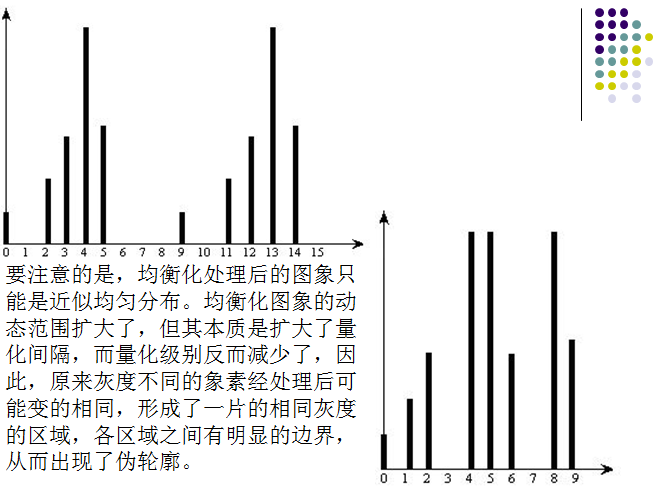代码中有详细注释如下：
[cpp] view plain
1. // ID_DYS_JHH:表示图像均衡化 相见算法
2. void CImageProcessingView::OnDysJhh()
3. {
4.     if(numPicture==0) {
5.         AfxMessageBox("载入图片后才能图像均衡化!",MB_OK,0);
6.         return;
7.     }
8.     AfxMessageBox("图像均衡化!",MB_OK,0);
9.
10.     //第一步:获取图像的数据信息
11.     //此操作可以在打开图片时就进行 在直方图采样(ZFTCY)中也有该代码
12.     FILE *fpo = fopen(BmpName,"rb");
15.
16.     int i,j,k;
17.     for(j=0;j<256;j++) { //定义数组并清零
18.         Red[j]=0;
19.         Green[j]=0;
20.         Blue[j]=0;
21.     }
22.
23.     //计算4个数据
24.     unsigned char red,green,blue;
25.     int IntRed,IntGreen,IntBlue;                  //强制转换
26.     double sumRedHD=0,sumGreenHD=0,sumBlueHD=0;   //记录像素总的灰度值和
27.     for(i=0; i<m_nImage/3; i++ )
28.     {
30.         IntRed=int(red);
31.         sumRedHD=sumRedHD+IntRed;
32.         if( IntRed>=0 && IntRed<256 ) Red[IntRed]++;
33.
35.         IntGreen=int(green);
36.         sumGreenHD=sumGreenHD+IntGreen;
37.         if( IntGreen>=0 && IntGreen<256 ) Green[IntGreen]++;
38.
40.         IntBlue=int(blue);
41.         sumBlueHD=sumBlueHD+IntBlue;
42.         if( IntBlue>=0 && IntBlue<256 ) Blue[IntBlue]++;
43.     }
44.     fclose(fpo);
45.
46.     /*****************************************************************/
47.     /* 图像均衡化处理
48.     /* 利用全局变量 Red Blue Green
49.     /* 第一步:用3个数组Count..记录0-255灰度出现的概率，即
50.     /*        概率=该灰度出现次数*3/总得像素 (因为分成3部分RGB)
51.     /* 第二步:i从1开始，令s[i]=s[i]+s[i-1] 记录新概率数
52.     /* 第三步:用一个数组L记录新的调色板索引值，即
53.     /*        L[i]=s[i]×(256-1)结果四舍五入2.8即为3
54.     /* 第四步:将老的索引值对应的概率合并，作为对应的新的索引值的概率
55.     /*   1.原来的索引值0,1都对应了新的索引值0，则灰度索引值为0的概率
56.     /*     为P0+P1=0.03
57.     /*   2.新的索引值3和7找不到老的索引值与之对应，所以令Q3和Q7为0
58.     /*****************************************************************/
59.
60.     //记录出现的概率,会加到1 用于相加到调色板
61.     float CountRed,CountGreen,CountBlue;
62.     //记录原始数据,不会相加到1 用于计算新灰度概率
63.     float CountRedLin,CountGreenLin,CountBlueLin;
64.
65.     for( k=0 ; k<256 ; k++ )
66.     {
67.         CountRed[k]=(float)(Red[k])*3/m_nImage;
68.         CountRedLin[k]=CountRed[k];
69.         CountGreen[k]=(float)(Green[k])*3/m_nImage;
70.         CountGreenLin[k]=CountGreen[k];
71.         CountBlue[k]=(float)(Blue[k])*3/m_nImage;
72.         CountBlueLin[k]=CountBlue[k];
73.     }
74.
75.     for( k=1 ; k<256 ; k++ )
76.     {
77.         CountRed[k]=CountRed[k]+CountRed[k-1];
78.         CountGreen[k]=CountGreen[k]+CountGreen[k-1];
79.         CountBlue[k]=CountBlue[k]+CountBlue[k-1];
80.     }
81.
82.     /****************************************************/
83.     /* 此处百度到一个四舍五入浮点型的算法:
84.     /* float a=3.456;   保留到小数点后两位
85.     /* float b=(int)((a * 100) + 0.5) / 100.0;
86.     /* output b=3.46
87.     /****************************************************/
88.
89.     int LRed,LGreen,LBlue;   //记录调色板
90.     for( k=0 ; k<256 ; k++ )
91.     {
92.         LRed[k]=(int)(CountRed[k]*(256-1)+0.5);
93.         LGreen[k]=(int)(CountGreen[k]*(256-1)+0.5);
94.         LBlue[k]=(int)(CountBlue[k]*(256-1)+0.5);
95.     }
96.
97.     //第三步:处理均衡化图像写入 打开临时的图片
98.     fpo = fopen(BmpName,"rb");
101.
102.     FILE *fpw = fopen(BmpNameLin,"wb+");
105.
106.     //m_nWidth*m_nHeight 读取图片最后一行不为m_nWidth时会报错 改为m_nImage/3
107.     for( i=0; i<m_nImage/3 ; i++ )
108.     {
112.
113.         red=LRed[int(red)];
114.         green=LGreen[int(green)];
115.         blue=LBlue[int(blue)];
116.
117.         fwrite(&red,sizeof(char),1,fpw);
118.         fwrite(&green,sizeof(char),1,fpw);
119.         fwrite(&blue,sizeof(char),1,fpw);
120.     }
121.     fclose(fpw);
122.     numPicture = 2;
123.     level=101;
124.     Invalidate();
125. }
运行结果如下图所示，图像增强而且异常清晰：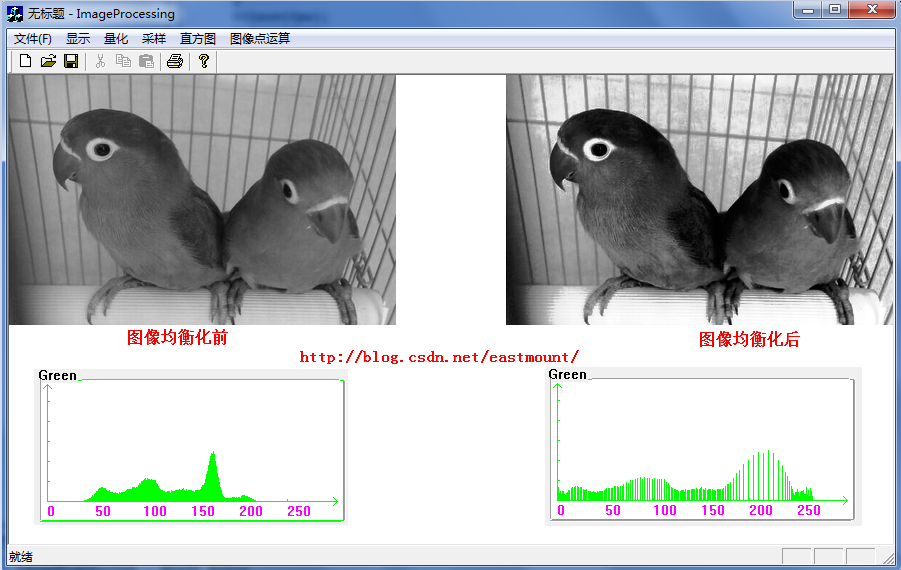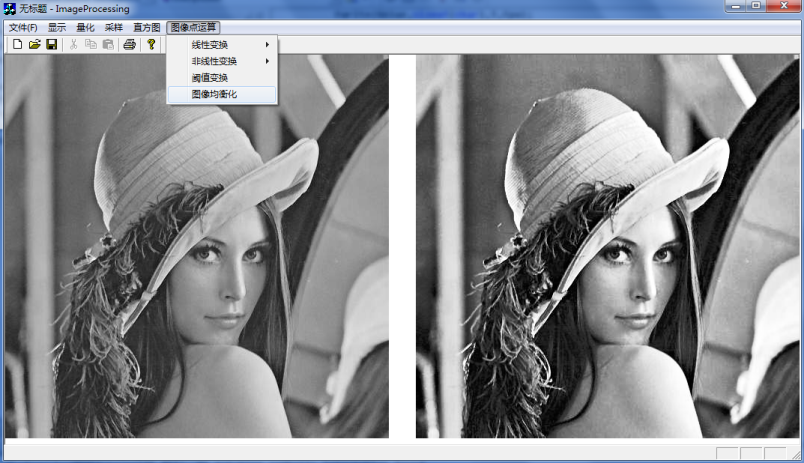最后介绍下图像对比度拉伸，它就是把你感兴趣的灰度范围拉开，使得该范围内像素，亮的更亮，暗的更暗，从而达到增强对比度的目的。
如下图所示，a、b、c为三段直线的斜率，g1old和g2old表示途中要进行对比度扩展的范围，g1new和g2new表示对应的新值。当g1old=g2old就是二值图像阈值化处理。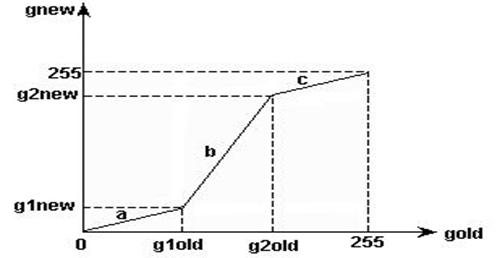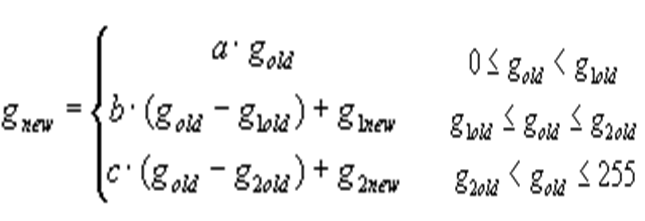由于灰度界别也是255这个约束，所以满足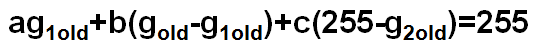其中g1old=100，g2old=150，b=3.0的运行效果如下所示：## 基于c++的灰度阈值变换

2014-10-16 15:47:37 u014033518 阅读数 1000
• ###### matlab 基于阈值分割的区域生长的医学图像分割3讲

matlab 基于阈值分割的区域生长的医学图像分割3讲 </p>

3课时 23分钟 614人学习 刘昱显
免费试看

y=（x>T）?255:0               T：阈值

LPSTR  lpDIBBits;	//指向源DIB图像的指针
long	lWidth;		//源图像宽度
long	lHeight;	//源图像高度
BYTE	T;			//阈值

//------------------------------

for(int i=0;i<lHeight;i++)
for(int j=0;j<lWidth;j++)
{
lpSrc=(unsighned char *)lpDIBBits+lLineBytes*(lHeight-1-i)+j;//lpDIBBits[i][j]
if(DB>T)
*lpSrc=255;
else
*lpSrc=0;
}//for_j

## [Python图像处理] 二十二.Python图像傅里叶变换原理及实现

2019-04-23 16:24:29 Eastmount 阅读数 19609
• ###### matlab 基于阈值分割的区域生长的医学图像分割3讲

matlab 基于阈值分割的区域生长的医学图像分割3讲 </p>

3课时 23分钟 614人学习 刘昱显
免费试看

PS：请求帮忙点个Star，哈哈，第一次使用Github，以后会分享更多代码，一起加油。

1.图像傅里叶变换
2.Numpy实现傅里叶变换
3.Numpy实现傅里叶逆变换
4.OpenCV实现傅里叶变换
5.OpenCV实现傅里叶逆变换

PS：文章参考自己以前系列图像处理文章及OpenCV库函数，同时参考如下文献：
《数字图像处理》（第3版），冈萨雷斯著，阮秋琦译，电子工业出版社，2013年.
《数字图像处理学》（第3版），阮秋琦，电子工业出版社，2008年，北京.
《OpenCV3编程入门》，毛星云，冷雪飞，电子工业出版社，2015，北京.

tenderwx-数字图像处理-傅里叶变换在图像处理中的应用

# 一.图像傅里叶变换原理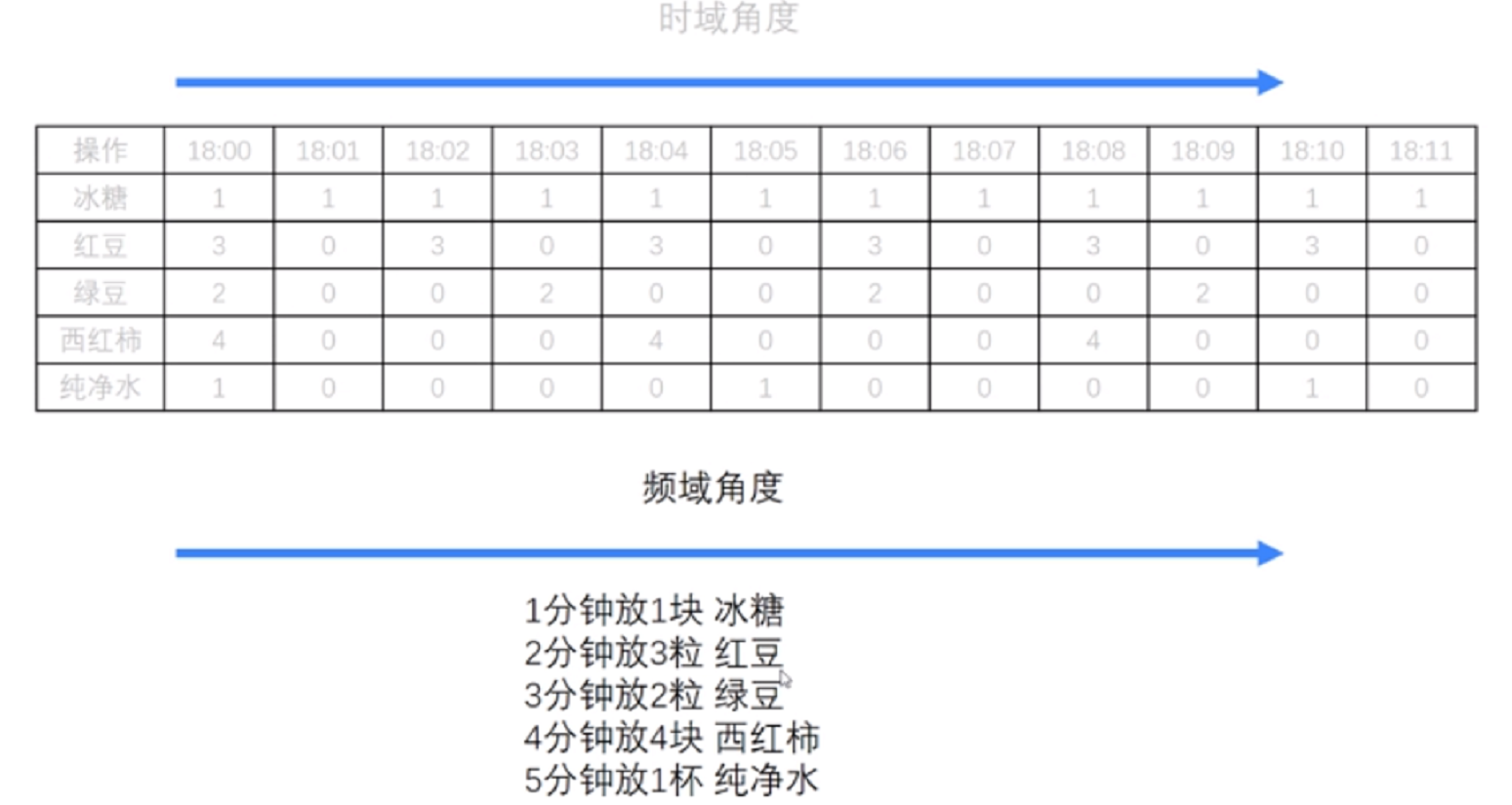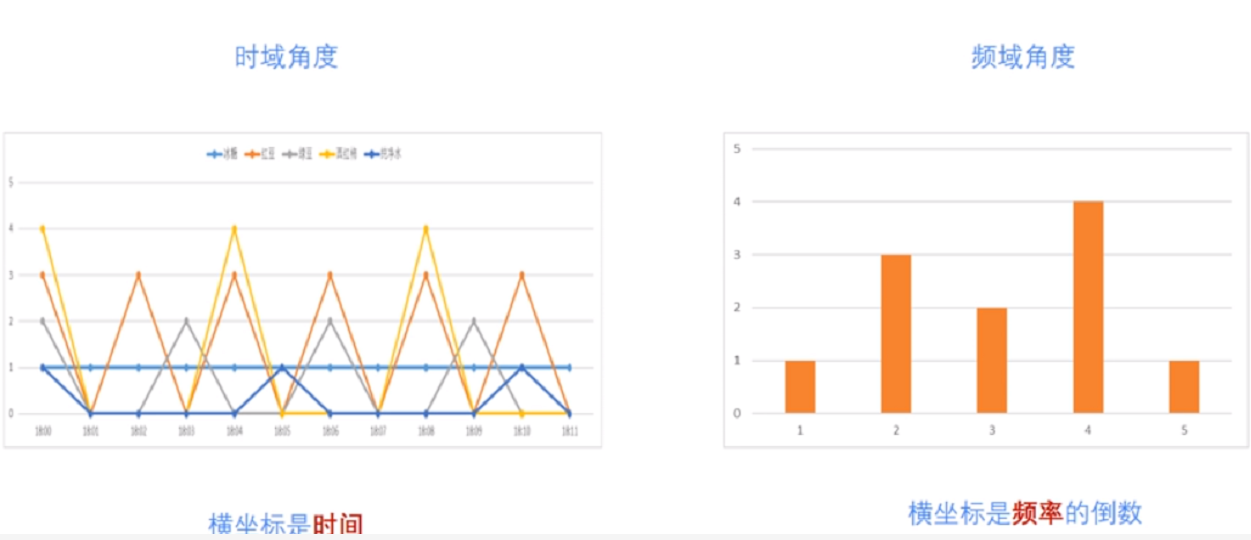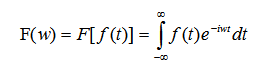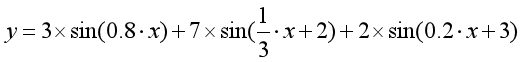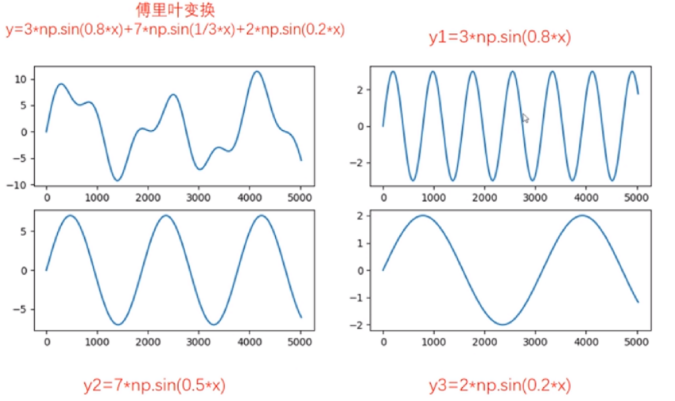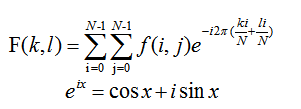# 二.Numpy实现傅里叶变换

Numpy中的 FFT包提供了函数 np.fft.fft2()可以对信号进行快速傅里叶变换，其函数原型如下所示，该输出结果是一个复数数组（Complex Ndarry）。

fft2(a, s=None, axes=(-2, -1), norm=None)

• a表示输入图像，阵列状的复杂数组
• s表示整数序列，可以决定输出数组的大小。输出可选形状（每个转换轴的长度），其中s表示轴0，s表示轴1。对应fit(x,n)函数中的n，沿着每个轴，如果给定的形状小于输入形状，则将剪切输入。如果大于则输入将用零填充。如果未给定’s’，则使用沿’axles’指定的轴的输入形状
• axes表示整数序列，用于计算FFT的可选轴。如果未给出，则使用最后两个轴。“axes”中的重复索引表示对该轴执行多次转换，一个元素序列意味着执行一维FFT
• norm包括None和ortho两个选项，规范化模式（请参见numpy.fft）。默认值为无

Numpy中的fft模块有很多函数，相关函数如下：

#计算一维傅里叶变换
numpy.fft.fft(a, n=None, axis=-1, norm=None)
#计算二维的傅里叶变换
numpy.fft.fft2(a, n=None, axis=-1, norm=None)
#计算n维的傅里叶变换
numpy.fft.fftn()
#计算n维实数的傅里叶变换
numpy.fft.rfftn()
#返回傅里叶变换的采样频率
numpy.fft.fftfreq()
#将FFT输出中的直流分量移动到频谱中央
numpy.fft.shift()

# -*- coding: utf-8 -*-
import cv2 as cv
import numpy as np
from matplotlib import pyplot as plt

#读取图像

#快速傅里叶变换算法得到频率分布
f = np.fft.fft2(img)

#默认结果中心点位置是在左上角,
#调用fftshift()函数转移到中间位置
fshift = np.fft.fftshift(f)

#fft结果是复数, 其绝对值结果是振幅
fimg = np.log(np.abs(fshift))

#展示结果
plt.subplot(121), plt.imshow(img, 'gray'), plt.title('Original Fourier')
plt.axis('off')
plt.subplot(122), plt.imshow(fimg, 'gray'), plt.title('Fourier Fourier')
plt.axis('off')
plt.show()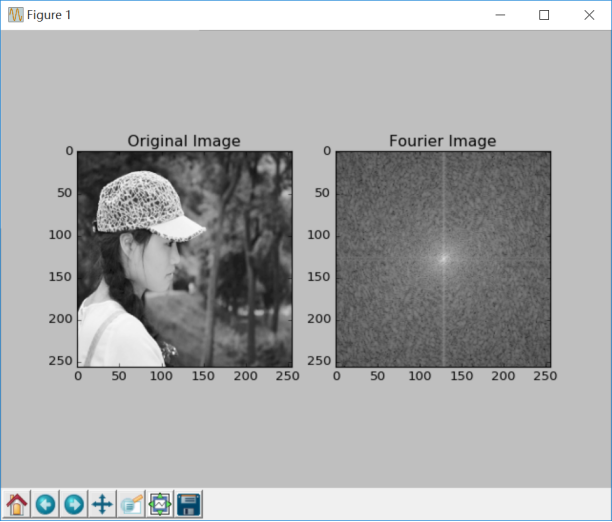# 三.Numpy实现傅里叶逆变换

#实现图像逆傅里叶变换，返回一个复数数组
numpy.fft.ifft2(a, n=None, axis=-1, norm=None)
#fftshit()函数的逆函数，它将频谱图像的中心低频部分移动至左上角
numpy.fft.fftshift()
#将复数转换为0至255范围
iimg = numpy.abs(逆傅里叶变换结果)

# -*- coding: utf-8 -*-
import cv2 as cv
import numpy as np
from matplotlib import pyplot as plt

#读取图像

#傅里叶变换
f = np.fft.fft2(img)
fshift = np.fft.fftshift(f)
res = np.log(np.abs(fshift))

#傅里叶逆变换
ishift = np.fft.ifftshift(fshift)
iimg = np.fft.ifft2(ishift)
iimg = np.abs(iimg)

#展示结果
plt.subplot(131), plt.imshow(img, 'gray'), plt.title('Original Image')
plt.axis('off')
plt.subplot(132), plt.imshow(res, 'gray'), plt.title('Fourier Image')
plt.axis('off')
plt.subplot(133), plt.imshow(iimg, 'gray'), plt.title('Inverse Fourier Image')
plt.axis('off')
plt.show()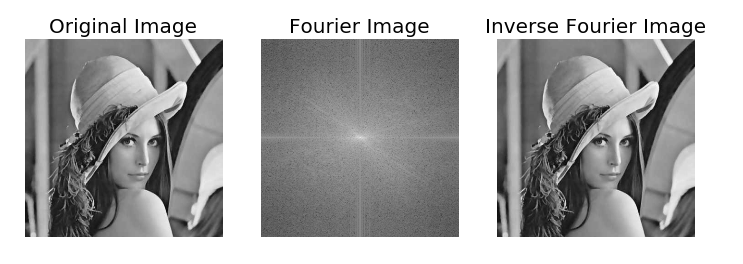# 四.OpenCV实现傅里叶变换

OpenCV 中相应的函数是cv2.dft()和用Numpy输出的结果一样，但是是双通道的。第一个通道是结果的实数部分，第二个通道是结果的虚数部分，并且输入图像要首先转换成 np.float32 格式。其函数原型如下所示：

dst = cv2.dft(src, dst=None, flags=None, nonzeroRows=None)

• src表示输入图像，需要通过np.float32转换格式
• dst表示输出图像，包括输出大小和尺寸
• flags表示转换标记，其中DFT _INVERSE执行反向一维或二维转换，而不是默认的正向转换；DFT _SCALE表示缩放结果，由阵列元素的数量除以它；DFT _ROWS执行正向或反向变换输入矩阵的每个单独的行，该标志可以同时转换多个矢量，并可用于减少开销以执行3D和更高维度的转换等；DFT _COMPLEX_OUTPUT执行1D或2D实数组的正向转换，这是最快的选择，默认功能；DFT _REAL_OUTPUT执行一维或二维复数阵列的逆变换，结果通常是相同大小的复数数组，但如果输入数组具有共轭复数对称性，则输出为真实数组
• nonzeroRows表示当参数不为零时，函数假定只有nonzeroRows输入数组的第一行（未设置）或者只有输出数组的第一个（设置）包含非零，因此函数可以处理其余的行更有效率，并节省一些时间；这种技术对计算阵列互相关或使用DFT卷积非常有用

cv2.magnitude(x, y)

• x表示浮点型X坐标值，即实部
• y表示浮点型Y坐标值，即虚部
最终输出结果为幅值，即：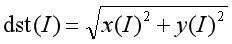# -*- coding: utf-8 -*-
import numpy as np
import cv2
from matplotlib import pyplot as plt

#读取图像

#傅里叶变换
dft = cv2.dft(np.float32(img), flags = cv2.DFT_COMPLEX_OUTPUT)

#将频谱低频从左上角移动至中心位置
dft_shift = np.fft.fftshift(dft)

#频谱图像双通道复数转换为0-255区间
result = 20*np.log(cv2.magnitude(dft_shift[:,:,0], dft_shift[:,:,1]))

#显示图像
plt.subplot(121), plt.imshow(img, cmap = 'gray')
plt.title('Input Image'), plt.xticks([]), plt.yticks([])
plt.subplot(122), plt.imshow(result, cmap = 'gray')
plt.title('Magnitude Spectrum'), plt.xticks([]), plt.yticks([])
plt.show()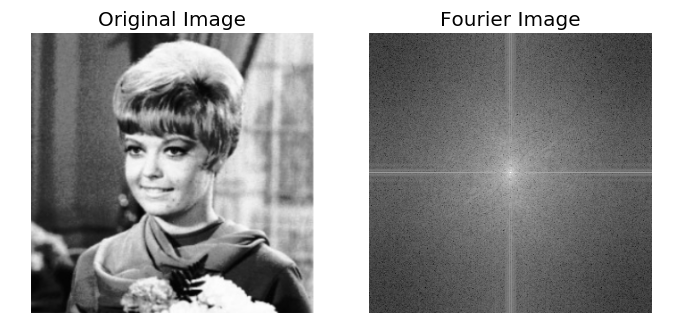# 五.OpenCV实现傅里叶逆变换

dst = cv2.idft(src[, dst[, flags[, nonzeroRows]]])

• src表示输入图像，包括实数或复数
• dst表示输出图像
• flags表示转换标记
• nonzeroRows表示要处理的dst行数，其余行的内容未定义（请参阅dft描述中的卷积示例）

# -*- coding: utf-8 -*-
import numpy as np
import cv2
from matplotlib import pyplot as plt

#读取图像

#傅里叶变换
dft = cv2.dft(np.float32(img), flags = cv2.DFT_COMPLEX_OUTPUT)
dftshift = np.fft.fftshift(dft)
res1= 20*np.log(cv2.magnitude(dftshift[:,:,0], dftshift[:,:,1]))

#傅里叶逆变换
ishift = np.fft.ifftshift(dftshift)
iimg = cv2.idft(ishift)
res2 = cv2.magnitude(iimg[:,:,0], iimg[:,:,1])

#显示图像
plt.subplot(131), plt.imshow(img, 'gray'), plt.title('Original Image')
plt.axis('off')
plt.subplot(132), plt.imshow(res1, 'gray'), plt.title('Fourier Image')
plt.axis('off')
plt.subplot(133), plt.imshow(res2, 'gray'), plt.title('Inverse Fourier Image')
plt.axis('off')
plt.show()# 六.总结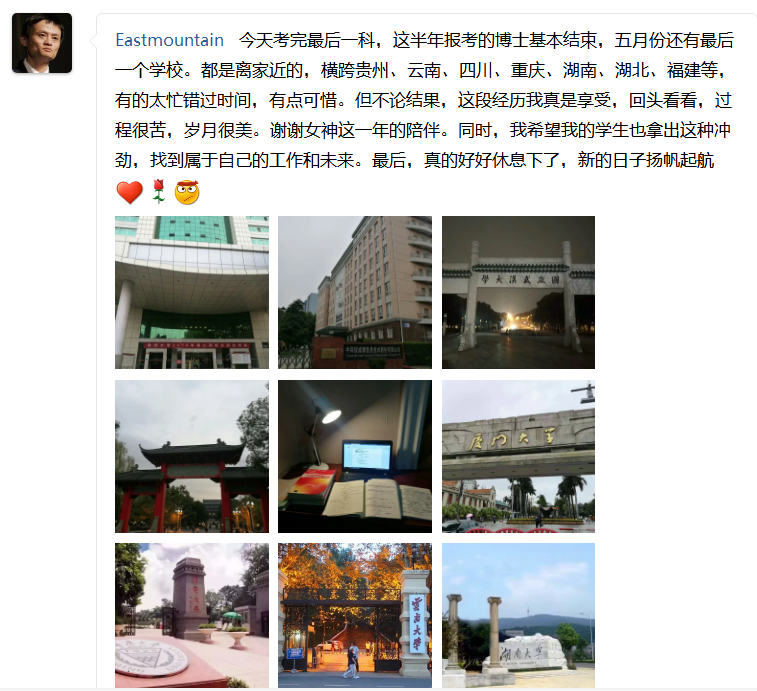（By：Eastmount 2019-04-23 周二下午6点写于花溪 https://blog.csdn.net/Eastmount )

## MATLAB图像的阈值变换

2019-01-08 18:45:02 dyq1995 阅读数 1470
• ###### matlab 基于阈值分割的区域生长的医学图像分割3讲

matlab 基于阈值分割的区域生长的医学图像分割3讲 </p>

3课时 23分钟 614人学习 刘昱显
免费试看

1、打开MATLAB软件，在其主界面的编辑器中写入下列代码：

I=imread('G:\MATLAB\bm.bmp');    %读取当前路径下的图片
BW=im2bw(I,map,0.4);        %阈值变换中设置的阈值数为0.4
imshow(I,map)
figure,imshow(BW)

2、保存代码至自定义路径下，点击运行，结果如下：